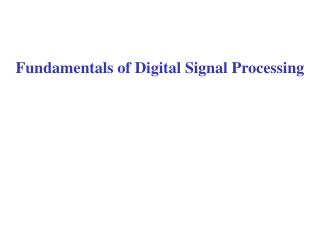DownloadDownload PresentationFundamentals of Digital Signal Processing

# Fundamentals of Digital Signal Processing

Télécharger la présentation## Fundamentals of Digital Signal Processing

- - - - - - - - - - - - - - - - - - - - - - - - - - - E N D - - - - - - - - - - - - - - - - - - - - - - - - - - -
##### Presentation Transcript

1. Fundamentals of Digital Signal Processing

2. Fourier Transform of continuous time signals with t in sec and F in Hz (1/sec). Examples:

3. Discrete Time Fourier Transform of sampled signals with f the digital frequency (no dimensions). Example: since, using the Fourier Series,

4. Property of DTFT • f is the digital frequency and has no dimensions • is periodic with period f = 1. • we only define it on one period

5. Sampled Complex Exponential: no aliasing 1. No Aliasing digital frequency

6. Sampled Complex Exponential: aliasing 2. Aliasing digital frequency

7. Mapping between Analog and Digital Frequency

8. Example • Then: • analog frequency • FT: • digital frequency • DTFT: for

9. Example • Then: • analog frequency • FT: • digital frequency • DTFT: for

10. Example • Then: • analog frequencies • FT: • digital frequencies • DTFT

11. Linear Time Invariant (LTI) Systems and z-Transform If the system is LTI we compute the output with the convolution: If the impulse response has a finite duration, the system is called FIR (Finite Impulse Response):

12. Z-Transform Facts: Frequency Response of a filter:

13. Digital Filters Ideal Low Pass Filter constant magnitude in passband… … and linear phase passband

14. Impulse Response of Ideal LPF Assume zero phase shift, This has Infinite Impulse Response, non recursive and it is non-causal. Therefore it cannot be realized.

15. Non Ideal Ideal LPF The good news is that for the Ideal LPF

16. Frequency Response of the Non Ideal LPF ripple attenuation stop pass stop transition region • LPF specified by: • passband frequency • passband ripple or • stopband frequency • stopband attenuation or

17. Best Design tool for FIR Filters: the Equiripple algorithm (or Remez). It minimizes the maximum error between the frequency responses of the ideal and actual filter. ripple attenuation impulse response Linear Interpolation

18. The total impulse response length N+1 depends on: • transition region • attenuation in the stopband Example: we want Passband: 3kHz Stopband: 3.5kHz Attenuation: 60dB Sampling Freq: 15 kHz Then: from the specs We determine the order the filter

19. Frequency response N=82 N=98

20. Example: Low Pass Filter Passband f = 0.2 Stopband f = 0.25 with attenuation 40dB Choose order N=40/(22*(0.25-0.20))=37 Almost 40dB!!!

21. Example: Low Pass Filter Passband f = 0.2 Stopband f = 0.25 with attenuation 40dB Choose order N=40 > 37 OK!!!

22. General FIR Filter of arbitrary Frequency Response Weights for Error: Then apply: … and always check frequency response if it is what you expect!

23. Example: for fp=0:0.01:0.2; % vector of passband frequencies fs=[0.25,0.5]; % stopband frequencies M=[1./sinc(fp), 0, 0]; % desired magnitudes Df=0.25-0.2; % transition region N=ceil(A/(22*Df)); % first guess of order h=firpm(N, [ fp, fs]/0.5,M); % impulse response

24. not very good here!

25. To improve it: • Increase order • Add weights w=[1*ones(1,length(fp)/2), 0.2*ones(1, length(fs)/2)]; h=firpm(N, [fp, fs]/0.5,M,w);